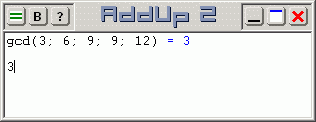Statistical operations with AddUp are performed with a set of functions that apply to a list of numbers or arbitrary length. Statistical functions provide summary information on the content of the list. Each function accepts a lists of arguments containing as many numbers as needed to represent a group under statistical study.Statistical functions include:

avg or mean
Returns the average (or mean) of a list of values. It returns 0 on an empty list, or the average value if the list is not empty. The average or mean is the sum of all values in a list divided by the item count.
avgG
Returns the geometric average of a list of values. It returns 0 on an empty list, or the geometric average value if the list is not empty. The geometric average of a list of values is the result of adding the square of all values (eg. using the sum2 function) and extracting the 'count' root of this sum.
count
Returns the count of values in a list (the total number of items). It returns 0 on an empty list, or the number of arguments if the list is not empty.
gcd or gcf
Returns the greatest common denominator (greatest common factor) of all values in a list. It returns 0 on an empty list, or the greatest common denominator of all values if the list is not empty.
lcm
Returns the least common multiple of all values in a list. It returns 0 on an empty list, or the least common multiple of all values if the list is not empty.
max
Returns the maximum value contained in a list. It returns 0 on an empty list, or the value of the largest argument if the list is not empty.
median
Returns the median of a list of values. It returns 0 on an empty list, or the median value if the list is not empty. The median value is the value that is at the center position of a list after it is sorted. For lists containing an even number of values, it is the average of the values found at the two middle positions.
min
Returns the minimum value contained in a list. It returns 0 on an empty list, or the value of the smallest argument if the list is not empty.
prod
Returns the product of all values contained in a list. It returns 0 on an empty list, or the product of all values if the list is not empty.
sd
Returns the sample standard deviation of a list of values. It returns 0 on an empty list, or the sample standard deviation if the list is not empty.
sdP
Returns the population standard deviation of a list of values. It returns 0 on an empty list, or the population standard deviation if the list is not empty.
sum
Returns the sum of all values contained in a list. It returns 0 on an empty list, or the sum of all values if the list is not empty.
sum2
Returns the sum of the square of all values contained in a list. It returns 0 on an empty list, or the sum of the square of all values if the list is not empty.
var
Returns the sample variance of a list of values. It returns 0 on an empty list, or the sample variance if the list is not empty.
varP
Returns the population variance of a list of values.It returns 0 on an empty list, or the population variance if the list is not empty.